• 递归树
千次阅读 多人点赞
2022-05-01 19:22:02

递归可以用来描述分形。分形常用来描述自然界中许多不规范的、真实对象的数字图形。

### 递归的图形还能说明递归是如何工作的。以递归绘制图树为例，绘制树枝的过程如下：首先绘制一条直线，然后左转，绘图（递归分支），右转，绘制（递归分支），最后返回原来的直线。

(31条消息) python Turtle Graphics海龟绘图工具_kaituozhizzz的博客-CSDN博客

前面有写到python中海龟绘图的使用

这里我们用海龟绘图制作一个递归树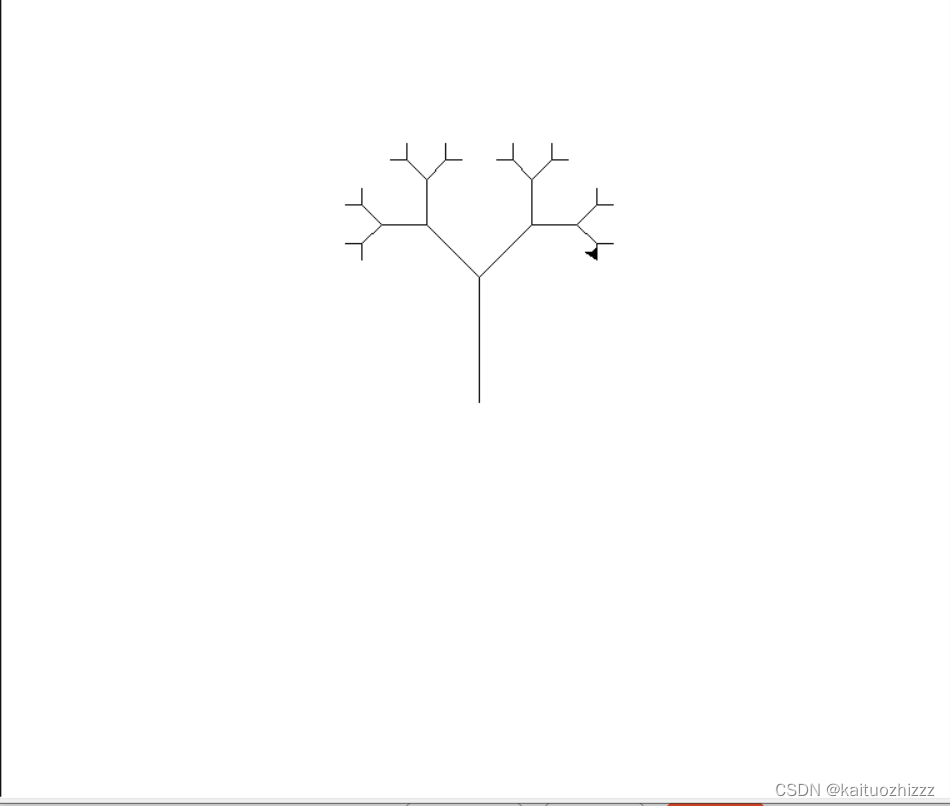# 上代码：

from turtle import *
def branch(length, level):
if level <= 0:
return
forward(length)
left(45)
branch(0.6*length, level-1)
right(90)
branch(0.6*length, level-1)
left(45)
backward(length)
return

left(90)
branch(100,5)

# Sierpinski三角形

绘制分形模式是一种有趣的递归实验，和前面一样，向前移动，递归调用，后转，左转120°。

需要跟踪层次，定义停止的基本情况，并且有一个长度参数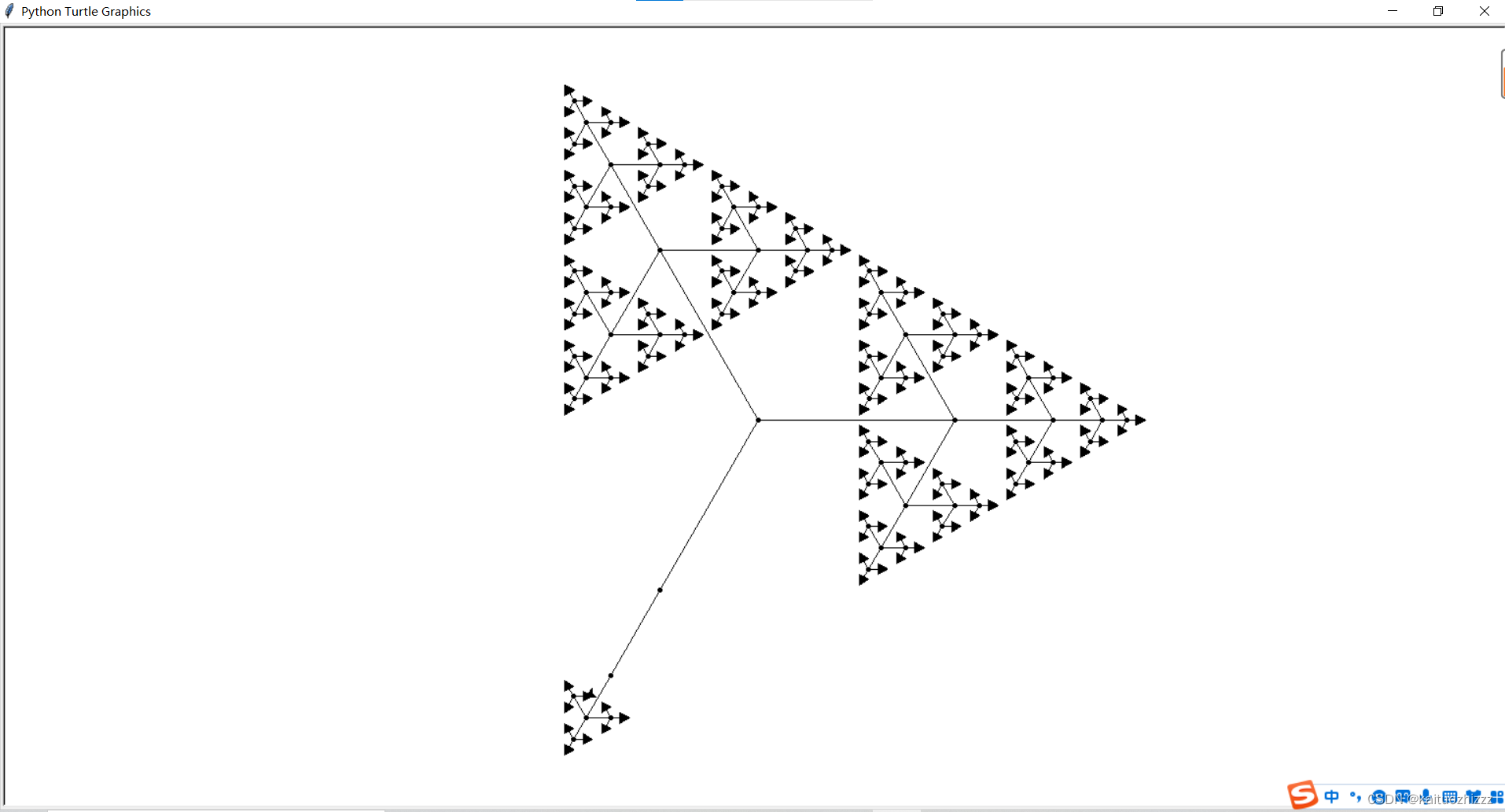# 上代码：

from turtle import *

def sierpinski(length,depth):
if depth > 1: dot()
if depth == 0:
stamp()
else:
forward(length)
sierpinski(length/2, depth-1)
backward(length)
left(120)
forward(length)
sierpinski(length/2, depth-1)
backward(length)
left(120)
forward(length)
sierpinski(length/2, depth-1)
backward(length)
left(120)

sierpinski(200,6)

###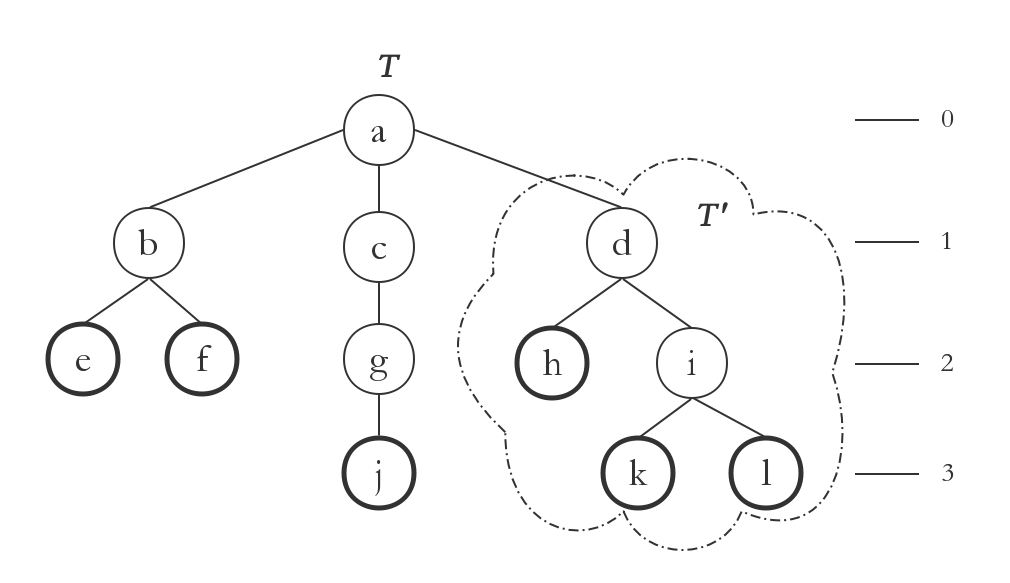树-Tree是一种数据结构，它用于模拟真实世界中的树形结构，通常描绘成上图的样子。为了说明方便，作者把每个节点用字母作了标识。

​ T是一棵树，树上的每个圆圈称之为一个节点-node，其中，a是树T的根节点 - root node。节点可以有后代 - descendents，其中，直接的后代称为儿子节点 - child。上图中，b,c,d是节点a的儿子，h,i是节点d的儿子。有儿子的节点称为内节点，没有儿子的节点称为叶子-leaf。上图中，e,f,k等节点没有儿子，作者用粗线圆作了标识，它们是叶子。

​ T’是树T的一棵子树-sub tree，其根节点为d，我们称树T’是树T中以d节点为根的子树。理论上，任何一个节点其及全部后代都可视为一棵树。

Point类有成员x和y，表明一个坐标点。TreeNode类表示一个树节点。类成员maxDepth表示树所允许的最大深度。类成员nBranches表示“建议”的树分叉数，即一根树支将分出多少根下层树支。此处之所以用了“建议”一词，是因为实际树的生成过程中分支数会引入随机成分。

​ TreeNode对象的children属性是其儿子列表，depth表示该节点在树中的深度。TreeNode对象的另外几个属性需要结合下图来理解，这是作者在树上取下的一个小树支。下图中，a是一个TreeNode节点，其bottom表示以该节点为根的子树的“下”顶点；其top表示以该节点为根的子树的“上”顶点。注意，这里的上下打了引号，那是因为实践中，树支因地球引力，可能事实上倒垂向下。

​ drawingTop定义为该节点自身（不含其子孙）的描绘用“上”顶点。a节点在树中的实际表现为一段树支，树支的描绘范围即为bottom至drawingTop点。从drawingTop一直到top则是a的子孙们的描绘区域。下图中，b,c,d为a的儿子，b,c,d也是TreeNode类型，其bottom应等于a的drawingTop。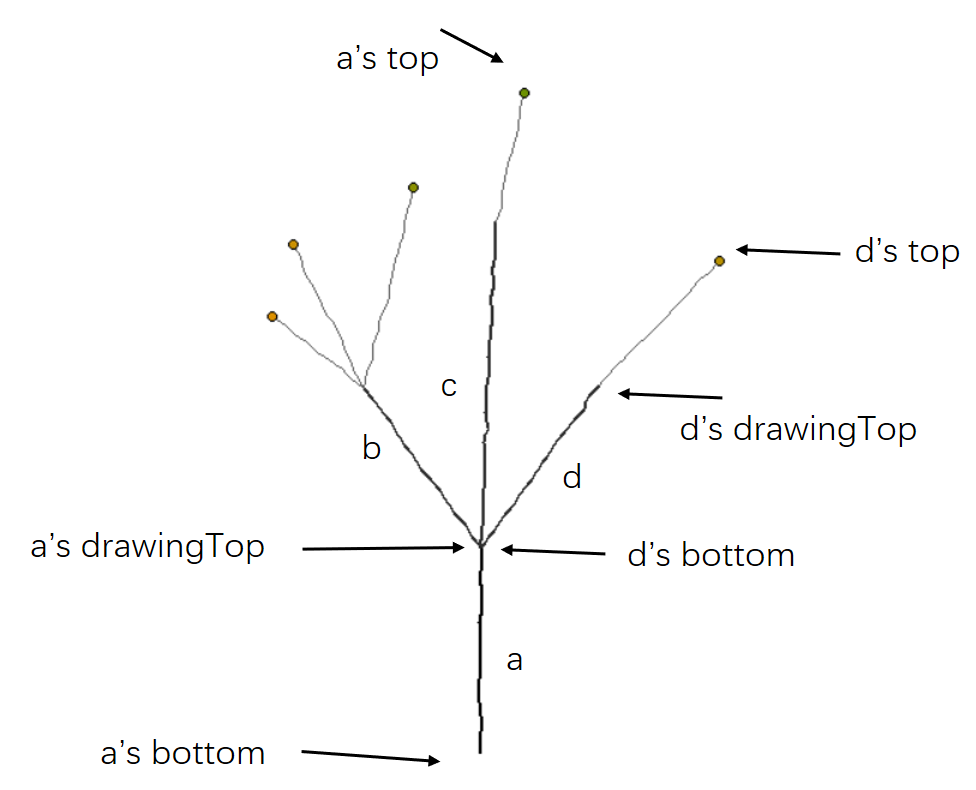### 总结：

递归得到本质是函数的自我调用，

# --------------------------------------------------------------------------------------------------------------------------------总结

递归的本质是，函数的自我调用，通常把一个大型复杂的问题层层转化为一个与原问题相似的规模较小的问题来求解，大大地减少了程序的代码量。

递归的能力在于用有限的语句来定义对象的无限集合

首先是使用了IF语句，然后自我调用也就是建立栈，最后函数回溯

希望多得几个赞啊，得个五一勋章算法 python 线性回归
更多相关内容
• 确定性均匀递归树的网络性质的研究已经有了大量的结果。关于确定性均匀递归树的谱的研究，章忠志等也提出了其拉普拉斯矩阵特征值的迭代关系。基于这个结果，我们又提出了推广的拉普拉斯矩阵，通过对其特征值进行分析...
• 在复杂网络的模型构建与性质研究领域中， 确定性均匀递归树网络模型DURT（Deterministic Uniform Recursive Tree）得到了广泛应用。在DURT网络模型的基础上提出一种适用范围更广的广义确定性均匀递归树演化模型GDURT...
• 深入浅出讲解借助递归树来分析递归算法的时间复杂度的方法，实例分析快排的时间复杂度，面对任何代码的时间复杂度分析，做到游刃有余、毫不畏惧。

# 递归树：如何借助树来求解递归算法的时间复杂度

今天，我们来讲这种数据结构的一种特殊应用，递归树。

我们都知道，递归代码的时间复杂度分析起来很麻烦。我们在《排序》那里讲过，如何利用递推公式，求解归并排序、快速排序的时间复杂度，但是，有些情况，比如快排的平均时间复杂度的分析，用递推公式的话，会涉及非常复杂的数学推导。

除了用递推公式这种比较复杂的分析方法，有没有更简单的方法呢？今天，我们就来学习另外一种方法，借助递归树来分析递归算法的时间复杂度。

## 递归树与时间复杂度分析

我们前面讲过，递归的思想就是，将大问题分解为小问题来求解，然后再将小问题分解为小小问题。这样一层一层地分解，直到问题的数据规模被分解得足够小，不用继续递归分解为止。

如果我们把这个一层一层的分解过程画成图，它其实就是一棵树。我们给这棵树起一个名字，叫作递归树。我这里画了一棵斐波那契数列的递归树，你可以看看。节点里的数字表示数据的规模，一个节点的求解可以分解为左右子节点两个问题的求解。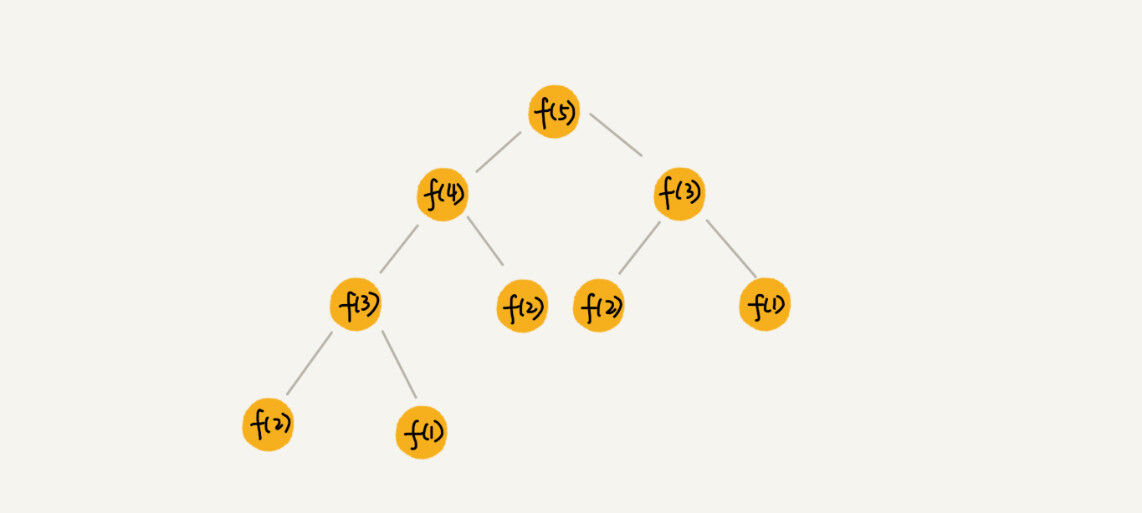通过这个例子，你对递归树的样子应该有个感性的认识了，看起来并不复杂。现在，我们就来看，如何用递归树来求解时间复杂度。

归并排序算法你还记得吧？它的递归实现代码非常简洁。现在我们就借助归并排序来看看，如何用递归树，来分析递归代码的时间复杂度。

归并排序的原理我就不详细介绍了，如果你忘记了，可以回看一下第 12 节的内容。归并排序每次会将数据规模一分为二。我们把归并排序画成递归树，就是下面这个样子：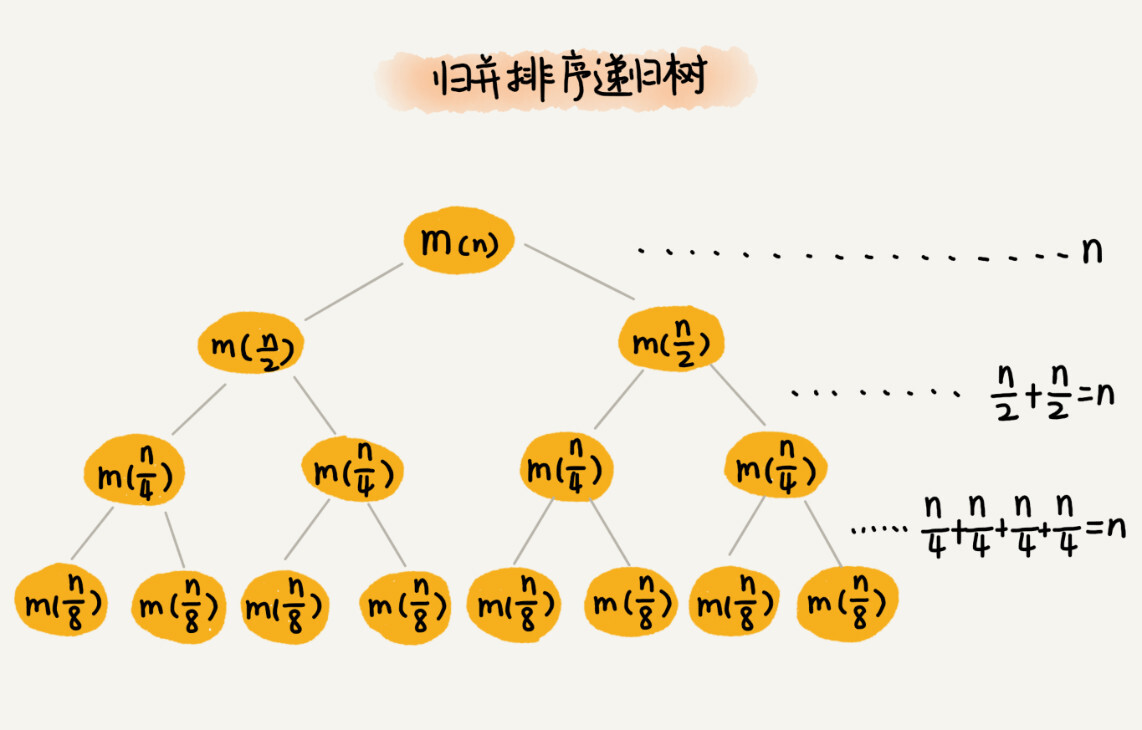因为每次分解都是一分为二，所以代价很低，我们把时间上的消耗记作常量 1。归并算法中比较耗时的是归并操作，也就是把两个子数组合并为大数组。从图中我们可以看出，每一层归并操作消耗的时间总和是一样的，跟要排序的数据规模有关。我们把每一层归并操作消耗的时间记作 n。

现在，我们只需要知道这棵树的高度 h，用高度 h 乘以每一层的时间消耗 n，就可以得到总的时间复杂度 O(n∗h)。

从归并排序的原理和递归树，可以看出来，归并排序递归树是一棵满二叉树。我们前两节中讲到，满二叉树的高度大约是 log2n，所以，归并排序递归实现的时间复杂度就是 O(nlogn)。我这里的时间复杂度都是估算的，对树的高度的计算也没有那么精确，但是这并不影响复杂度的计算结果。

利用递归树的时间复杂度分析方法并不难理解，关键还是在实战，所以，接下来我会通过三个实际的递归算法，带你实战一下递归的复杂度分析。学完这节课之后，你应该能真正掌握递归代码的复杂度分析。

## 实战一：分析快速排序的时间复杂度

在用递归树推导之前，我们先来回忆一下用递推公式的分析方法。你可以回想一下，当时，我们为什么说用递推公式来求解平均时间复杂度非常复杂？

快速排序在最好情况下，每次分区都能一分为二，这个时候用递推公式 T(n)=2T(n/2)+n，很容易就能推导出时间复杂度是 O(nlogn)。但是，我们并不可能每次分区都这么幸运，正好一分为二。

我们假设平均情况下，每次分区之后，两个分区的大小比例为 1:k。当 k=9 时，如果用递推公式的方法来求解时间复杂度的话，递推公式就写成 T(n)=T(n/10)+T(9n/10)+n。

这个公式可以推导出时间复杂度，但是推导过程非常复杂。那我们来看看，用递归树来分析快速排序的平均情况时间复杂度，是不是比较简单呢？

我们还是取 k 等于 9，也就是说，每次分区都很不平均，一个分区是另一个分区的 9 倍。如果我们把递归分解的过程画成递归树，就是下面这个样子：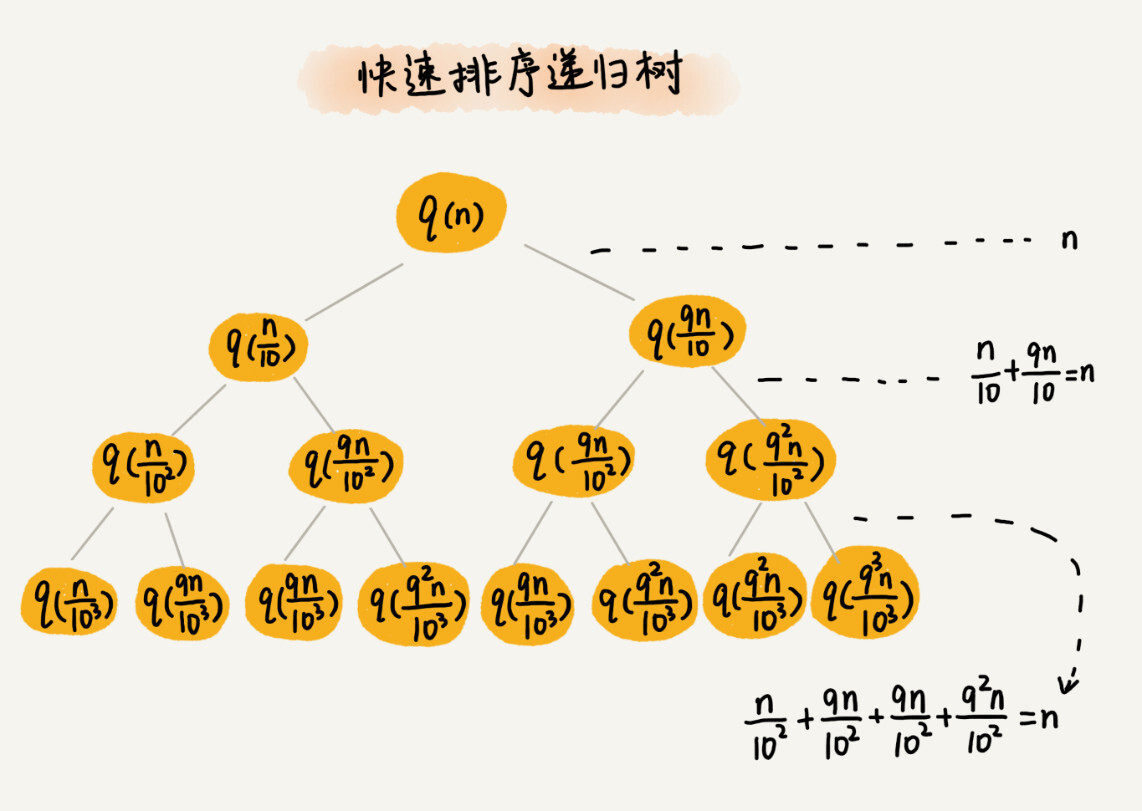快速排序的过程中，每次分区都要遍历待分区区间的所有数据，所以，每一层分区操作所遍历的数据的个数之和就是 n。我们现在只要求出递归树的高度 h，这个快排过程遍历的数据个数就是 h∗n ，也就是说，时间复杂度就是 O(h∗n)。

因为每次分区并不是均匀地一分为二，所以递归树并不是满二叉树。这样一个递归树的高度是多少呢？

我们知道，快速排序结束的条件就是待排序的小区间，大小为 1，也就是说叶子节点里的数据规模是 1。从根节点 n 到叶子节点 1，递归树中最短的一个路径每次都乘以 1/10，最长的一个路径每次都乘以 9/10。通过计算，我们可以得到，从根节点到叶子节点的最短路径是 log10n，最长的路径是 log10/9n。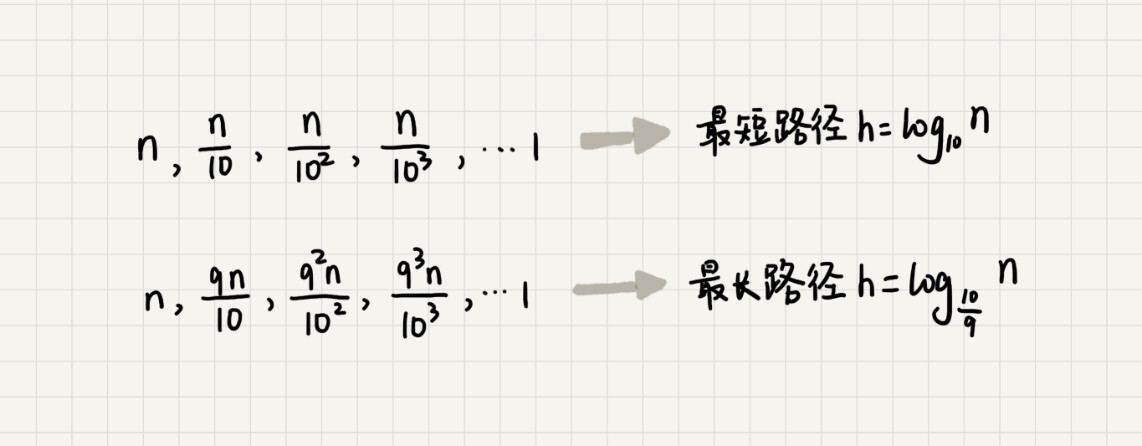所以，遍历数据的个数总和就介于 nlog10n 和 nlog10/9n 之间。根据复杂度的大 O 表示法，对数复杂度的底数不管是多少，我们统一写成 logn，所以，当分区大小比例是 1:9 时，快速排序的时间复杂度仍然是 O(nlogn)。

刚刚我们假设 k=9，那如果 k=99，也就是说，每次分区极其不平均，两个区间大小是 1:99，这个时候的时间复杂度是多少呢？

我们可以类比上面 k=9 的分析过程。当 k=99 的时候，树的最短路径就是 log100n，最长路径是 log100/99n，所以总遍历数据个数介于 nlog100n 和 nlog100/99n 之间。尽管底数变了，但是时间复杂度也仍然是 O(nlogn)。

也就是说，对于 k 等于 9，99，甚至是 999，9999……，只要 k 的值不随 n 变化，是一个事先确定的常量，那快排的时间复杂度就是 O(nlogn)。所以，从概率论的角度来说，快排的平均时间复杂度就是 O(nlogn)。

## 实战二：分析斐波那契数列的时间复杂度

在递归那一节中，我们举了一个跨台阶的例子，你还记得吗？那个例子实际上就是一个斐波那契数列。为了方便你回忆，我把它的代码实现贴在这里。

int f(int n) {
if (n == 1) return 1;
if (n == 2) return 2;
return f(n-1) + f(n-2);
}


这样一段代码的时间复杂度是多少呢？你可以先试着分析一下，然后再来看，我是怎么利用递归树来分析的。

我们先把上面的递归代码画成递归树，就是下面这个样子：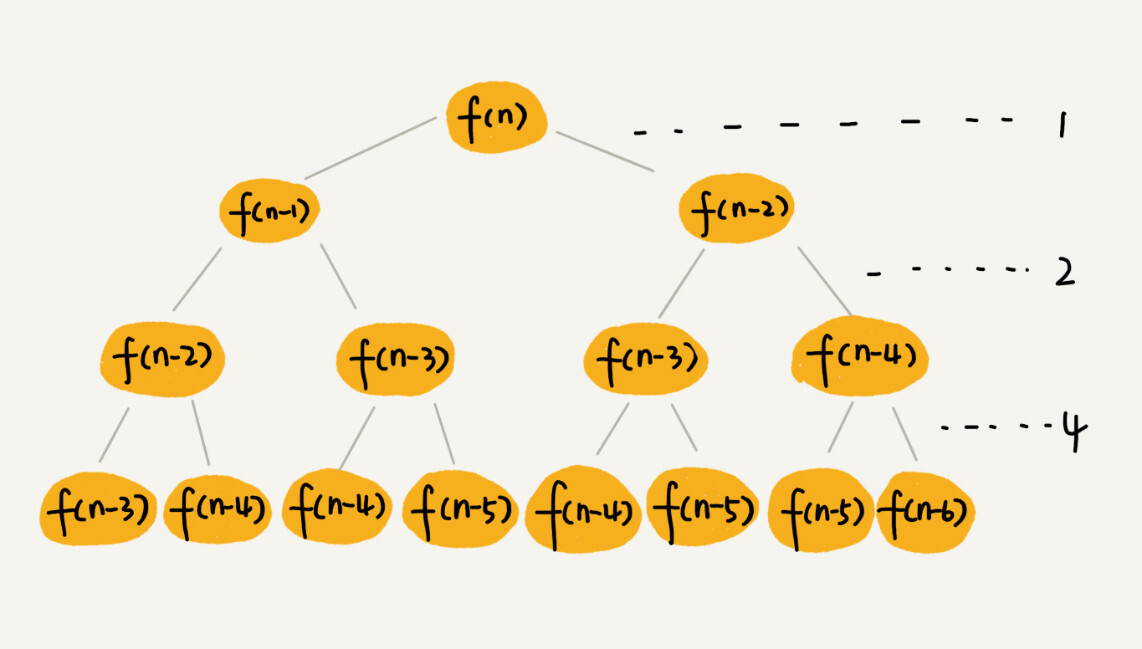这棵递归树的高度是多少呢？

f(n) 分解为 f(n−1) 和 f(n−2)，每次数据规模都是 −1 或者 −2，叶子节点的数据规模是 1 或者 2。所以，从根节点走到叶子节点，每条路径是长短不一的。如果每次都是 −1，那最长路径大约就是 n；如果每次都是 −2，那最短路径大约就是n/2。

每次分解之后的合并操作只需要一次加法运算，我们把这次加法运算的时间消耗记作 1。所以，从上往下，第一层的总时间消耗是 1，第二层的总时间消耗是 2，第三层的总时间消耗就是 22。依次类推，第 k 层的时间消耗就是 2k−1，那整个算法的总的时间消耗就是每一层时间消耗之和。

如果路径长度都为 n，那这个总和就是 2n−1如果路径长度都是 n/2 ，那整个算法的总的时间消耗就是 2n/2−1。所以，这个算法的时间复杂度就介于 O(2n) 和 O(2n/2) 之间。虽然这样得到的结果还不够精确，只是一个范围，但是我们也基本上知道了上面算法的时间复杂度是指数级的，非常高。

## 实战三：分析全排列的时间复杂度

前面两个复杂度分析都比较简单，我们再来看个稍微复杂的。

我们在高中的时候都学过排列组合。“如何把 n 个数据的所有排列都找出来”，这就是全排列的问题。

我来举个例子。比如，1，2，3 这样 3 个数据，有下面这几种不同的排列：

1, 2, 3
1, 3, 2
2, 1, 3
2, 3, 1
3, 1, 2
3, 2, 1

如何编程打印一组数据的所有排列呢？这里就可以用递归来实现。

如果我们确定了最后一位数据，那就变成了求解剩下 n−1 个数据的排列问题。而最后一位数据可以是 n 个数据中的任意一个，因此它的取值就有 n 种情况。所以，“n 个数据的排列”问题，就可以分解成 n 个“n−1 个数据的排列”的子问题。

如果我们把它写成递推公式，就是下面这个样子：

假设数组中存储的是1，2， 3…n。

f(1,2,…n) = {最后一位是1, f(n-1)} + {最后一位是2, f(n-1)} +…+{最后一位是n, f(n-1)}。

如果我们把递推公式改写成代码，就是下面这个样子：

// 调用方式：
// int[]a = a={1, 2, 3, 4}; printPermutations(a, 4, 4);
// k表示要处理的子数组的数据个数
public void printPermutations(int[] data, int n, int k) {
if (k == 1) {
for (int i = 0; i < n; ++i) {
System.out.print(data[i] + " ");
}
System.out.println();
}

for (int i = 0; i < k; ++i) {
int tmp = data[i];
data[i] = data[k-1];
data[k-1] = tmp;

printPermutations(data, n, k - 1);

tmp = data[i];
data[i] = data[k-1];
data[k-1] = tmp;
}
}


如果不用我前面讲的递归树分析方法，这个递归代码的时间复杂度会比较难分析。现在，我们来看下，如何借助递归树，轻松分析出这个代码的时间复杂度。

首先，我们还是画出递归树。不过，现在的递归树已经不是标准的二叉树了。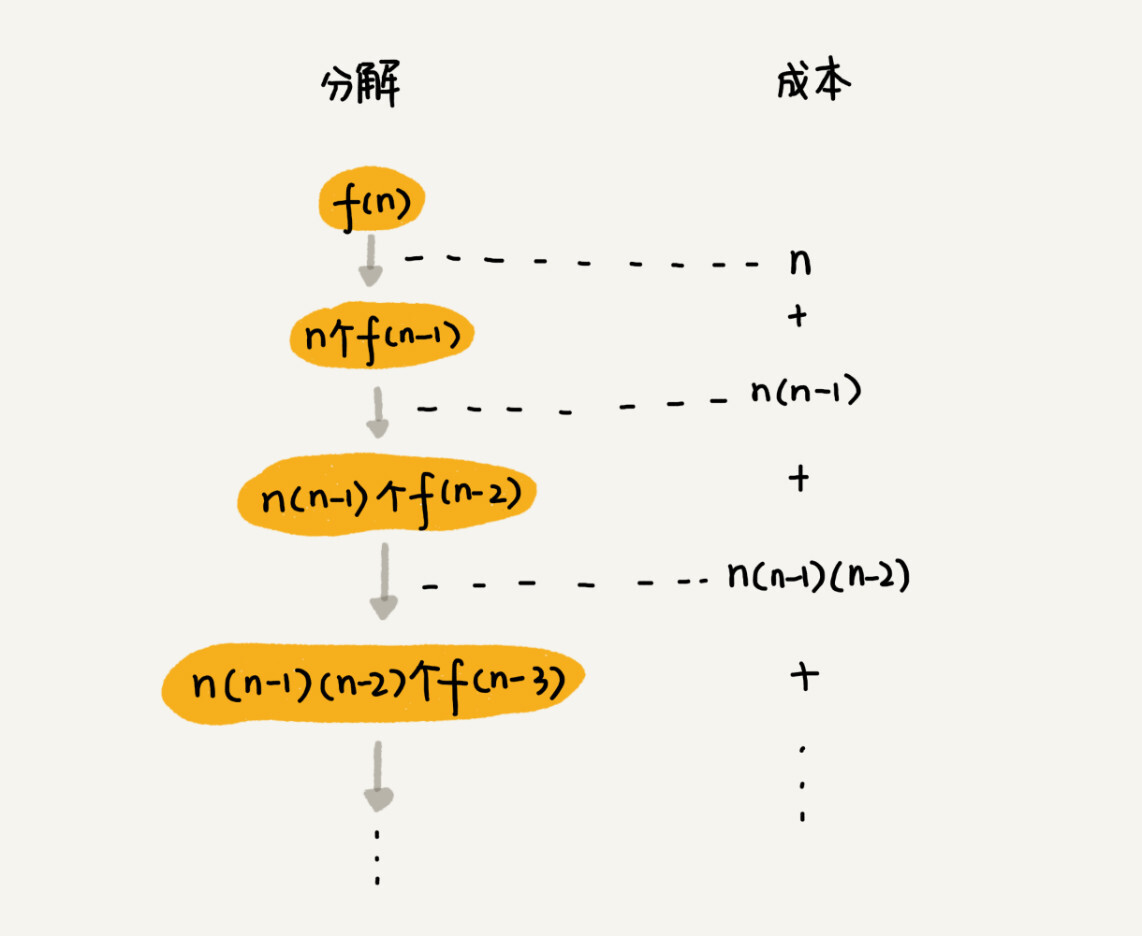第一层分解有 n 次交换操作，第二层有 n 个节点，每个节点分解需要 n−1 次交换，所以第二层总的交换次数是 n∗(n−1)。第三层有 n ∗ (n−1) 个节点，每个节点分解需要 n−2 次交换，所以第三层总的交换次数是 n ∗ (n−1) ∗ (n−2)。

以此类推，第 k 层总的交换次数就是 n ∗ (n−1) ∗ (n−2) ∗ … ∗ (n−k+1)。最后一层的交换次数就是 n∗(n−1) ∗ (n−2) ∗ … ∗ 2 ∗ 1。每一层的交换次数之和就是总的交换次数。

n + n * (n-1) + n * (n-1) * (n-2) +… + n * (n-1) * (n-2) * … * 2 * 1

这个公式的求和比较复杂，我们看最后一个数，n ∗ (n−1) ∗ (n−2) ∗ … ∗ 2 ∗ 1 等于 n!，而前面的 n−1 个数都小于最后一个数，所以，总和肯定小于 n ∗ n!，也就是说，全排列的递归算法的时间复杂度大于 O(n!)，小于 O(n ∗ n!)，虽然我们没法知道非常精确的时间复杂度，但是这样一个范围已经让我们知道，全排列的时间复杂度是非常高的。

这里我稍微说下，掌握分析的方法很重要，思路是重点，不要纠结于精确的时间复杂度到底是多少。

## 内容小结

今天，我们用递归树分析了递归代码的时间复杂度。加上我们在排序那一节讲到的递推公式的时间复杂度分析方法，我们现在已经学习了两种递归代码的时间复杂度分析方法了。

有些代码比较适合用递推公式来分析，比如归并排序的时间复杂度、快速排序的最好情况时间复杂度；有些比较适合采用递归树来分析，比如快速排序的平均时间复杂度。而有些可能两个都不怎么适合使用，比如二叉树的递归前中后序遍历。

时间复杂度分析的理论知识并不多，也不复杂，掌握起来也不难，但是，在我们平时的工作、学习中，面对的代码千差万别，能够灵活应用学到的复杂度分析方法，来分析现有的代码，并不是件简单的事情，所以，你平时要多实战、多分析，只有这样，面对任何代码的时间复杂度分析，你才能做到游刃有余、毫不畏惧。

展开全文算法 数据结构
• 用VB画植物, , 花, 藤蔓等等 也可以生成内摆线 支持配置文件和画面的保存 一个是直接EXE, 有VB的直接用, 另一个是安装版, 没VB的装
• 创建递归树之后，对树的每层的各子问题的代价进行求和，得到每一层的代价，然后将所有层的代价加起来，得到整棵递归树的总代价，这个总代价就是递归式的解。当然，递归树方法是一种粗略的方法，因为递归树会引入一些...

### 笔记

在应用代入法求解递归式时，需要事先做出一个好的猜测。然而，有时候做出好的猜测是很困难的，此时可以考虑采用递归树方法。在递归树中，每个结点表示一个单一子问题的代价。创建递归树之后，对树的每层的各子问题的代价进行求和，得到每一层的代价，然后将所有层的代价加起来，得到整棵递归树的总代价，这个总代价就是递归式的解。当然，递归树方法是一种粗略的方法，因为递归树会引入一些“不精确”因素，这一点在后文再详细介绍。如果要精确求解递归式，还需要用代入法对递归树得到的解进行验证。
以递归式 T ( n ) = 3 T ( ⌊ n / 4 ⌋ ) + Θ ( n 2 ) T(n)=3T(⌊n/4⌋)+Θ(n^2) 为例。为简化分析，我们将递归树中的 Θ ( n 2 ) Θ(n^2) 这一渐近符号项替换为一个代数式 c n 2 cn^2 。这里引入了第一个“不精确”因素，但是我们认为这不会影响最终结果。于是我们将递归树变为 T ( n ) = 3 T ( ⌊ n / 4 ⌋ ) + c n 2 T(n)=3T(⌊n/4⌋)+cn^2 。为方便起见，我们还假定 n n 4 4 的幂。这里又引入了一个“不精确”因素，但我们同样认为这不会影响最终结果。现在可以创建递归树，如下图所示。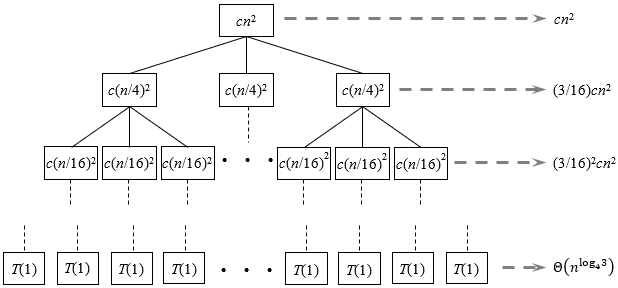可以看到，递归树每下降一层，子问题规模减少为上一层的 1 / 4 1/4 ，因此深度为 i i 的结点，其子问题的规模为 n / 4 i n/4^i 。当到达叶结点时，子问题规模减为 1 1 。假设叶结点深度为 k k ，那么有 n / 4 k = 1 n/4^k = 1 ，得到 k = l o g 4 n k={\rm log}_4n 。所以叶结点深度为 l o g 4 n {\rm log}_4n ，这也说明整棵递归树的高度为 l o g 4 n {\rm log}_4n
现在统计递归树每一层的代价。对深度为 i i 的非叶结点来说，每一个结点对应一个规模为 n / 4 i n/4^i 的子问题，每个子问题产生代价 c ( n / 4 i ) 2 = c n 2 / 1 6 i c(n/4^i)^2 = cn^2/16^i 。从递归树可以看到，递归树每下降一层，结点数目变为上一层的 3 3 倍，因此深度为 i i 的结点一共有 3 i 3^i 个。于是可以得到，除叶结点那一层外，深度为 i i 的那一层的结点代价之和为 3 i • ( c n 2 / 1 6 i ) = ( 3 / 16 ) i c n 2 3^i•(cn^2/16^i) = (3/16)^icn^2
现在考虑叶结点。一个叶结点对应规模为 1 1 的子问题，它产生的代价为 T ( 1 ) T(1) 。通常来说， T ( 1 ) T(1) 为常数时间，即 T ( 1 ) = Θ ( 1 ) T(1) = Θ(1) 。叶结点一共有 3 l o g 4 n = n l o g 4 3 3^{{\rm log}_4n}=n^{{\rm log}_43} 个，所以叶结点那一层的代价为 n l o g 4 3 • T ( 1 ) = Θ ( n l o g 4 3 ) n^{{\rm log}_43}•T(1)=Θ(n^{{\rm log}_43})
现在将递归树每一层代价加起来，得到递归树的总代价为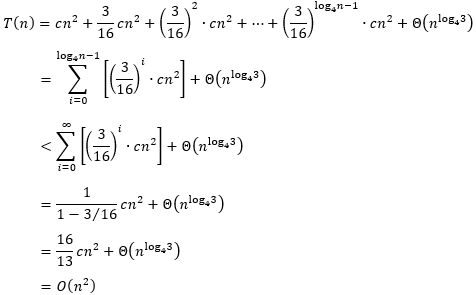这样，对原始递归式 T ( n ) = 3 T ( ⌊ n / 4 ⌋ ) + Θ ( n 2 ) T(n)=3T(⌊n/4⌋)+Θ(n^2) ，我们得到了一个猜测解 T ( n ) = O ( n 2 ) T(n)=O(n^2) 。由于根结点对总代价的贡献为 c n 2 cn^2 ，所以根结点的代价支配了整棵递归树的总代价。
现在用代入法对猜测解进行验证。我们要证明的是：存在正常数 d d ，使得 T ( n ) ≤ d n 2 T(n) ≤ dn^2 对足够大的 n n 都成立。将 T ( n ) ≤ d n 2 T(n) ≤ dn^2 代入递归式得到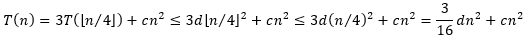当 d ≥ 16 13 c d ≥ \frac{16}{13}c 时，不等式 3 16 d n 2 + c n 2 ≤ d n 2 \frac{3}{16}dn^2+cn^2≤dn^2 成立，此时 T ( n ) ≤ d n 2 T(n) ≤ dn^2 成立。因此 T ( n ) = O ( n 2 ) T(n)=O(n^2) 得证。
现在考察一个更复杂的递归式 T ( n ) = T ( n / 3 ) + T ( 2 n / 3 ) + O ( n ) T(n)=T(n/3)+T(2n/3)+O(n) 。与之前一样，将渐近项 O ( n ) O(n) 替换为代数式 c n cn ，递归式变为 T ( n ) = T ( n / 3 ) + T ( 2 n / 3 ) + c n T(n)=T(n/3)+T(2n/3)+cn 。现在创建递归树，如下图所示。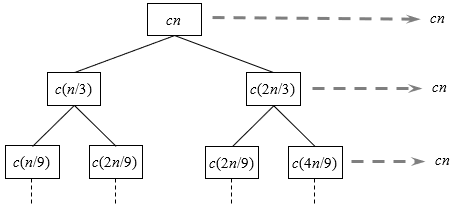由于每个结点的左右孩子的规模不一样，这意味着每个结点的左右子树的高度不一样，右子树比左子树要高，因此这棵树并不是一棵满二叉树。从根结点到叶结点的最长简单路径是 n → ( 2 / 3 ) n → ( 2 / 3 ) 2 n → … → 1 n → (2/3)n → (2/3)^2n → … → 1 。假设树的高度为 h h ，那么有 ( 2 / 3 ) h • n = 1 (2/3)^h•n = 1 ，得到 h = l o g 3 / 2 n h={\rm log}_{3/2}n
在上图中，我们只画出了递归树的顶部几层，可以看到每层的代价都为 c n cn 。然而由于递归树并不是一棵满二叉树，所以并不是每层的代价都为 c n cn 。随着递归树的层级越往下降，缺失的结点会越来多，这些存在缺失结点的层级的代价小于 c n cn 。只有递归树靠顶部的那些不存在缺失结点的层级，它们的代价等于 c n cn 。由于我们只需要通过递归树获得一个猜测解，所以我们不考虑这些缺失结点的影响，于是我们猜测解为 T ( n ) = O ( c n • l o g 3 / 2 n ) = O ( n l g n ) T(n)=O(cn•{\rm log}_{3/2}n)=O(n{\rm lg}n) 。注意，这里又引入了另一种形式的“不精确”因素。
现在用代入法对猜测解进行验证。我们要证明的是：存在正常数 d d ，使得 T ( n ) ≤ d n l g n T(n) ≤ dn{\rm lg}n 对足够大的 n n 都成立。将 T ( n ) ≤ d n l g n T(n) ≤ dn{\rm lg}n 代入递归式得到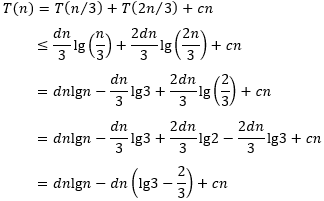只要取 d ≥ c l g 3 − 2 / 3 d≥\frac{c}{{\rm lg}3-2/3} ，就能使得不等式 d n l g n − d n ( l g 3 − 2 3 ) + c n ≤ d n l g n dn{\rm lg}n-dn({\rm lg}3-\frac{2}{3})+cn≤dn{\rm lg}n 成立，此时 T ( n ) ≤ d n l g n T(n)≤dn{\rm lg}n 成立。因此 T ( n ) = O ( n l g n ) T(n)=O(n{\rm lg}n) 得证。

### 练习

4.4-1 对递归式 T ( n ) = 3 T ( ⌊ n / 2 ⌋ ) + n T(n)=3T(⌊n/2⌋)+n ，利用递归树确定一个好的渐近上界，用代入法进行验证。

创建递归树，如下图所示。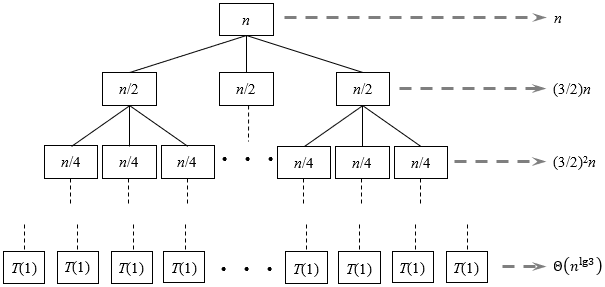在递归树中，深度为 i i 的结点对应规模为 n / 2 i n/2^i 的子问题。当 n / 2 i = 1 n/2^i = 1 时，即 i = l g n i = {\rm lg}n 时，子问题规模变为 1 1 ，这对应于叶结点 T ( 1 ) T(1) ，因此树的高度 h = l g n h = {\rm lg}n
每层的结点数都是上一层的 3 3 倍，因此深度为 i i 的结点数为 3 i 3^i 。深度为 i i 的结点对应的子问题规模为 n / 2 i n/2^i ，除叶结点外，深度为 i i 的每个结点的代价为 n / 2 i n/2^i 。因此，除叶结点外，深度为 i i 的所有结点的代价为 3 i • ( n / 2 i ) = ( 3 / 2 ) i n 3^i•(n/2^i )=(3/2)^in 。叶结点一共有 3 l g n = n l g 3 3^{{\rm lg}n}=n^{{\rm lg}3} 个，所以叶结点那一层的代价为 Θ ( n l g 3 ) Θ(n^{{\rm lg}3})
现在将每一层的代价加起来，得到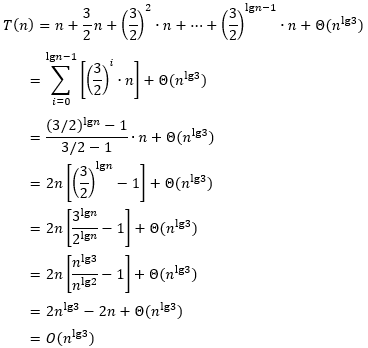下面用代入法验证这一结果。在做归纳假设时，需要减去一个低阶项，才能顺利完成证明。于是我们要证明的是：存在正常数 c c d d ，使得 T ( n ) ≤ c n l g 3 − d n T(n)≤cn^{{\rm lg}3}-dn 对足够大的n都成立。
取初始情况 T ( 1 ) = 1 T(1) = 1 。只要取 c ≥ d + 1 c ≥ d + 1 ，就能使得 T ( 1 ) ≤ c • 1 l g 3 − d • 1 = c − d T(1) ≤ c•1^{{\rm lg}3} − d•1 = c − d 成立。
现在考虑 n ≥ 2 n ≥ 2 的情况。假设 T ( n ) ≤ c n l g 3 − d n T(n)≤cn^{{\rm lg}3}-dn 1 , 2 , … , n − 1 1, 2, …, n−1 都成立，于是有
T ( n ) = 3 T ( ⌊ n / 2 ⌋ ) + n ≤ 3 c ( n 2 ) l g 3 − 3 d • n 2 + n = c n l g 3 − ( 3 2 d − 1 ) n T(n)=3T(⌊n/2⌋)+n≤3c(\frac{n}{2})^{{\rm lg}3}-3d•\frac{n}{2}+n=cn^{{\rm lg}3}-(\frac{3}{2}d-1)n
现在要选取合适的 c c d d ，使得不等式 c n l g 3 − ( 3 2 d − 1 ) n ≤ c n l g 3 − d n cn^{{\rm lg}3}-(\frac{3}{2}d-1)n≤cn^{{\rm lg}3}-dn 成立。对该不等式做一下变换。
c n l g 3 − ( 3 2 d − 1 ) n ≤ c n l g 3 − d n cn^{{\rm lg}3}-(\frac{3}{2}d-1)n≤cn^{{\rm lg}3}-dn 　　⇔　　 ( 3 2 d − 1 ) n ≥ d n (\frac{3}{2}d-1)n≥dn 　　⇔　　 d ≥ 2 d≥2
因此，只要取 d ≥ 2 d ≥ 2 ，并且 c c 取任意值，就能使能不等式 c n l g 3 − ( 3 2 d − 1 ) n ≤ c n l g 3 − d n cn^{{\rm lg}3}-(\frac{3}{2}d-1)n≤cn^{{\rm lg}3}-dn 成立，此时 T ( n ) ≤ c n l g 3 − d n T(n)≤cn^{{\rm lg}3}-dn 成立。
综合考虑初始情况 T ( 1 ) T(1) ，我们最终要取 d ≥ 2 d ≥ 2 并且 c ≥ d + 1 c ≥ d + 1 。于是 T ( n ) = O ( n l g 3 ) T(n)=O(n^{{\rm lg}3}) 得证。

4.4-2 对递归式 T ( n ) = T ( n / 2 ) + n 2 T(n)=T(n/2)+n^2 ，利用递归树确定一个好的渐近上界，用代入法进行验证。

创建递归树，如下图所示。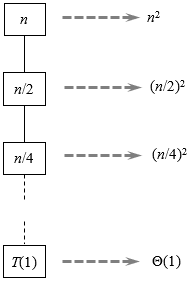在递归树中，深度为 i i 的结点对应规模为 n / 2 i n/2^i 的子问题。当 n / 2 i = 1 n/2^i = 1 时，即 i = l g n i = {\rm lg}n 时，子问题规模变为 1 1 ，这对应于叶结点 T ( 1 ) T(1) ，因此树的高度 h = l g n h = {\rm lg}n
每层只有一个结点。除叶结点外，深度为 i i 的结点的代价为 ( n / 2 i ) 2 = n 2 / 4 i (n/2^i)^2 = n^2/4^i 。叶结点的代价为 T ( 1 ) = Θ ( 1 ) T(1) = Θ(1) 。现在将每一层的代价加起来，得到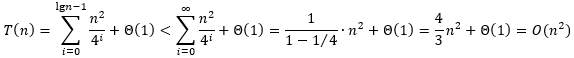用代入法验证这一结果比较简单，这里省略具体过程。

4.4-3 对递归式 T ( n ) = 4 T ( n / 2 + 2 ) + n T(n)=4T(n/2+2)+n ，利用递归树确定一个好的渐近上界，用代入法进行验证。

直接对递归式 T ( n ) = 4 T ( n / 2 + 2 ) + n T(n)=4T(n/2+2)+n 创建递归树很困难。将递归式变换为 T ( n ) = 4 T ( n / 2 ) + n T(n)=4T(n/2)+n ，因为当 n n 足够大时 n / 2 + 2 n/2+2 n / 2 n/2 很接近，我们预计这样做不会影响最终结果。现在创建递归树，如下图所示。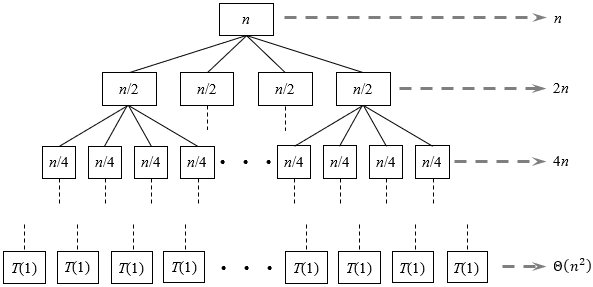在递归树中，深度为 i i 的结点对应规模为 n / 2 i n/2^i 的子问题。当 n / 2 i = 1 n/2^i = 1 时，即 i = l g n i = {\rm lg}n 时，子问题规模变为 1 1 ，这对应于叶结点 T ( 1 ) T(1) ，因此树的高度 h = l g n h = {\rm lg}n
每层的结点数都是上一层的 4 4 倍，因此深度为 i i 的结点数为 4 i 4^i 。深度为 i i 的结点对应的子问题规模为 n / 2 i n/2^i ，除叶结点外，深度为 i i 的每个结点的代价为 n / 2 i n/2^i 。因此，除叶结点外，深度为 i i 的所有结点的代价为 4 i • ( n / 2 i ) = 2 i • n 4^i•(n/2^i)=2^i•n 。叶结点一共有 4 l g n = n 2 4^{{\rm lg}n}=n^2 个，所以叶结点那一层的代价为 Θ ( n 2 ) Θ(n^2)
现在将每一层的代价加起来，得到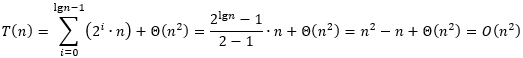下面用代入法验证这一结果。我们没有办法直接证明 T ( n ) ≤ c n 2 T(n)≤cn^2 ，不妨证明：存在正常数 c c d d ，使得 T ( n ) ≤ c ( n − 4 ) 2 − d ( n − 4 ) T(n)≤c(n-4)^2-d(n-4) 对足够大的 n n 都成立。
要使原递归式 T ( n ) = 4 T ( n / 2 + 2 ) + n T(n)=4T(n/2+2)+n 有意义，必须有 n > n / 2 + 2 n > n/2 + 2 ，得到 n > 4 n > 4 ，即 n ≥ 5 n ≥ 5 。所以取初始情况 T ( 5 ) = 1 T(5) = 1 。只要取 c ≥ d + 1 c ≥ d + 1 ，就能使得 T ( 5 ) ≤ c • ( 5 − 4 ) 2 − d • ( 5 − 4 ) = c − d T(5) ≤ c•(5−4)^2 − d•(5−4) = c − d 成立。
现在考虑 n ≥ 6 n ≥ 6 的情况。假设 T ( n ) ≤ c ( n − 4 ) 2 − d ( n − 4 ) T(n)≤c(n-4)^2-d(n-4) 5 , 6 , … , n − 1 5, 6, …, n−1 都成立，于是有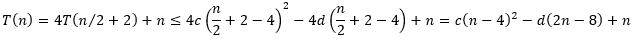现在要选取合适的 c c d d ，使得不等式 c ( n − 4 ) 2 − d ( 2 n − 8 ) + n ≤ c ( n − 4 ) 2 − d ( n − 4 ) c(n-4)^2-d(2n-8)+n≤c(n-4)^2-d(n-4) 成立。对这个不等式做一下变换。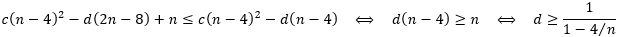当 n ≥ 6 n ≥ 6 时， 1 / ( 1 − 4 / n ) 1/(1-4/n) 是单调递减的，并且在 n = 6 n = 6 时取得最大值为 3 3 。因此，只要取 d ≥ 3 d ≥ 3 ，就能使得不等式 c ( n − 4 ) 2 − d ( 2 n − 8 ) + n ≤ c ( n − 4 ) 2 − d ( n − 4 ) c(n-4)^2-d(2n-8)+n≤c(n-4)^2-d(n-4) 成立，此时 T ( n ) ≤ c ( n − 4 ) 2 − d ( n − 4 ) T(n)≤c(n-4)^2-d(n-4) 成立。
综合考虑初始情况 T ( 5 ) T(5) ，我们最终要取 d ≥ 3 d ≥ 3 并且 c ≥ d + 1 c ≥ d + 1 。于是 T ( n ) = O ( n 2 ) T(n)=O(n^2) 得证。

4.4-4 对递归式 T ( n ) = 2 T ( n − 1 ) + 1 T(n)=2T(n-1)+1 ，利用递归树确定一个好的渐近上界，用代入法进行验证。

创建递归树，如下图所示。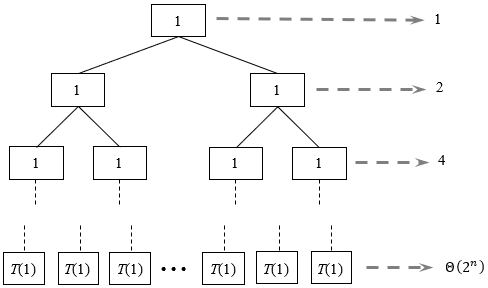在递归树中，深度为 i i 的结点对应规模为 n − i n − i 的子问题。当 n − i = 1 n − i = 1 时，即 i = n − 1 i = n − 1 时，子问题规模变为 1 1 ，这对应于叶结点 T ( 1 ) T(1) ，因此树的高度 h = n − 1 h = n − 1
每层的结点数都是上一层的 2 2 倍，因此深度为 i i 的结点数为 2 i 2^i 。除叶结点外，每个结点的代价都为 1 1 。因此，除叶结点外，深度为i的所有结点的代价为 2 i • 1 = 2 i 2^i•1=2^i 。叶结点一共有 2 n − 1 2^{n-1} 个，所以叶结点那一层的代价为 Θ ( 2 n ) Θ(2^n)
现在将每一层的代价加起来，得到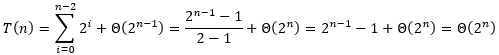下面用代入法验证这一结果。在做归纳假设时，需要减去一个低阶项，才能顺利完成证明。我们要证明的是：存在正常数 c c d d ，使得 T ( n ) ≤ c • 2 n − d T(n)≤c•2^n-d 对足够大的 n n 都成立。
取初始情况 T ( 1 ) = 1 T(1) = 1 。只要取 c ≥ ( d + 1 ) / 2 c ≥ (d+1)/2 ，就能使得 T ( 1 ) ≤ c • 2 1 – d = 2 c – d T(1) ≤ c•2^1 – d = 2c – d 成立。
现在考虑 n ≥ 2 n ≥ 2 的情况。假设 T ( n ) ≤ c • 2 n − d T(n)≤c•2^n-d 1 , 2 , … , n − 1 1, 2, …, n−1 都成立，于是有
T ( n ) = 2 T ( n − 1 ) + 1 ≤ 2 c • 2 n − 1 − 2 d + 1 = c • 2 n − 2 d + 1 T(n)=2T(n-1)+1≤2c•2^{n-1}-2d+1=c•2^n-2d+1
只要取 d ≥ 1 d ≥ 1 ，就能使得 c • 2 n − 2 d + 1 ≤ c • 2 n − d c•2^n-2d+1≤c•2^n-d 成立，此时 T ( n ) ≤ c • 2 n − d T(n)≤c•2^n-d 成立。
综合考虑初始情况 T ( 1 ) T(1) ，我们最终要取 d ≥ 1 d ≥ 1 并且 c ≥ ( d + 1 ) / 2 c ≥ (d+1)/2 。于是 T ( n ) = O ( 2 n ) T(n)=O(2^n) 得证。

4.4-5 对递归式 T ( n ) = T ( n − 1 ) + T ( n / 2 ) + n T(n)=T(n-1)+T(n/2)+n ，利用递归树确定一个好的渐近上界，用代入法进行验证。

创建递归树，如下图所示。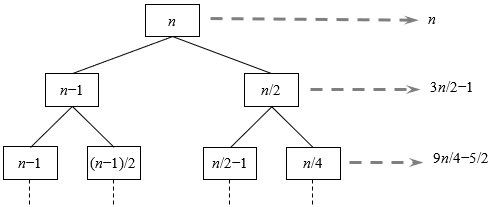该递归树显然不是一棵满二叉树。从根结点到叶结点的最短简单路径是 n → n / 2 → n / 4 → … → 1 n → n/2 → n/4 → … → 1 。假设这一最短简单路径上叶结点深度为 k k ，那么有 ( 1 / 2 ) k • n = 1 (1/2)k•n = 1 ，得到 k = l g n k={\rm lg}n 。从根结点到叶结点的最短简单路径是 n → n − 1 → n − 2 → … → 1 n → n−1 → n−2 → … → 1 。假设这一最短简单路径上叶结点深度为 l l ，那么有 n − l = 1 n − l = 1 ，得到 l = n − 1 l=n-1
从递归树可以看到，第i层的代价为 ( 3 / 2 ) i • n – e (3/2)^i•n – e ，其中 e e 为低阶项。我们要确定 T ( n ) T(n) 的渐近上界，为方便起见，忽略每一层代价中的低阶项 e e ，统计第 0 0 层到第 n − 1 n − 1 层的代价之和，得到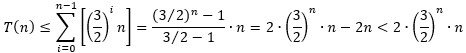当 n n 足够大时，有 2 • ( 3 / 2 ) n • n < 2 n 2•(3/2)^n•n<2^n ，因此得到 T ( n ) = O ( 2 n ) T(n) = O(2^n)
下面用代入法验证这一结果。在做归纳假设时，需要减去一个低阶项，才能顺利完成证明。我们要证明的是：存在正常数 c c d d ，使得 T ( n ) ≤ c • 2 n − d n T(n)≤c•2^n-dn 对足够大的n都成立。
取初始情况 T ( 1 ) = 1 T(1) = 1 。只要取 c ≥ ( d + 1 ) / 2 c ≥ (d+1)/2 ，就能使得 T ( 1 ) ≤ c • 2 1 – d • 1 = 2 c – d T(1) ≤ c•2^1 – d•1 = 2c – d 成立。
现在考虑 n ≥ 2 n ≥ 2 的情况。假设 T ( n ) ≤ c • 2 n − d n T(n)≤c•2^n-dn 1 , 2 , … , n − 1 1, 2, …, n−1 都成立，于是有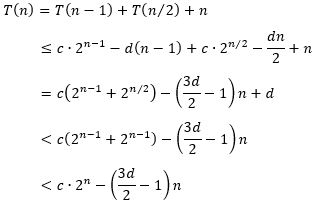现在要选取合适的 c c d d ，使得不等式 c • 2 n − ( 3 d 2 − 1 ) n ≤ c • 2 n − d n c•2^n-(\frac{3d}{2}-1)n≤c•2^n-dn 成立。对该不等式做一下变换。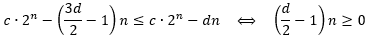显然，只要取 d ≥ 2 d ≥ 2 ，就能使得 c • 2 n − ( 3 d 2 − 1 ) n ≤ c • 2 n − d n c•2^n-(\frac{3d}{2}-1)n≤c•2^n-dn 成立，此时 T ( n ) ≤ c • 2 n − d n T(n)≤c•2^n-dn 成立。
综合考虑初始情况 T ( 1 ) T(1) ，我们最终要取 d ≥ 2 d ≥ 2 并且 c ≥ ( d + 1 ) / 2 c ≥ (d+1)/2 。于是 T ( n ) = O ( 2 n ) T(n)=O(2^n) 得证。

4.4-6 对递归式 T ( n ) = T ( n / 3 ) + T ( 2 n / 3 ) + c n T(n)=T(n/3)+T(2n/3)+cn ，利用递归树论证其解为 Ω ( n l g n ) Ω(n{\rm lg}n) ，其中 c c 为常数。

创建递归树，如下图所示。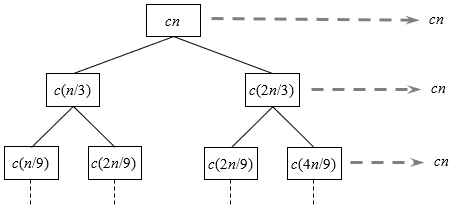由于每个结点的左右孩子的规模不一样，这意味着每个结的左右子树的高度不一样，右子树比左子树要高，因此这棵树并不是一棵满二叉树。从根结点到叶结点的最短简单路径是 n → ( 1 / 3 ) n → ( 1 / 3 ) 2 n → … → 1 n → (1/3)n → (1/3)^2n → … → 1 。假设这一最短简单路径上叶结点深度为 k k ，那么有 ( 1 / 3 ) k • n = 1 (1/3)^k•n = 1 ，得到 k = l o g 3 n k={\rm log}_3n 。这意味着递归树中第 l o g 3 n {\rm log}_3n 层及以上的层级都是满的，这些层级的每一层的代价都为 c n cn
我们求的是 T ( n ) T(n) 的渐近下界，因此可以只统计递归树中第 l o g 3 n {\rm log}_3n 层及以上的层级的代价。于是得到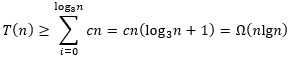用代入法验证这一结果比较简单，这里省略具体过程。

4.4-7 对递归式 T ( n ) = 4 T ( ⌊ n / 2 ⌋ ) + c n T(n)=4T(⌊n/2⌋)+cn c c 为常数），画出递归树，并给出其解的一个渐近紧确界。用代入法进行验证。

本题的递归树与练习4.4-3的递归树几乎一样，如下图所示。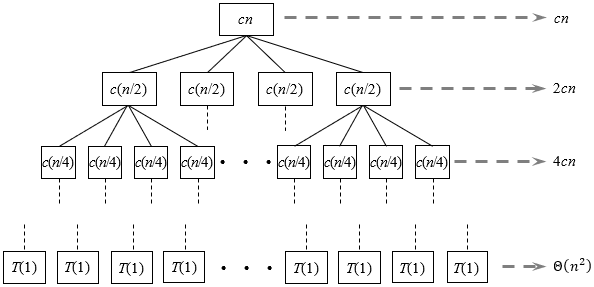将每一层的代价加起来，得到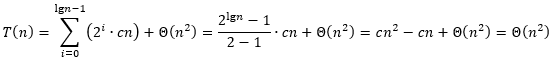下面用代入法验证这一结果。在做归纳假设时，需要减去一个低阶项，才能顺利完成证明。于是我们要证明的是：存在正常数 d 1 d_1 d 2 d_2 e e ，使得 d 1 ( n 2 − e n ) ≤ T ( n ) ≤ d 2 ( n 2 − e n ) d_1(n^2-en)≤T(n)≤d_2(n^2-en) 对足够大的 n n 都成立。需要分两步证明。
(1) 证明存在正常数 d 1 d_1 e e ，使得 T ( n ) ≥ d 1 ( n 2 − e n ) T(n)≥d_1(n^2-en) 对足够大的n都成立
取初始情况 T ( 1 ) = 1 T(1) = 1 。只要取 d 1 ≤ 1 / ( 1 − e ) d_1 ≤ 1/(1−e) 并且 e < 1 e < 1 ，就能使得 T ( 1 ) ≥ d 1 ( 1 2 − e • 1 ) = d 1 ( 1 − e ) T(1) ≥ d_1(1^2 − e•1) = d_1(1 − e) 成立。
现在考虑 n ≥ 2 n ≥ 2 的情况。假设 T ( n ) ≥ d 1 ( n 2 − e n ) T(n)≥d_1(n^2-en) 1 , 2 , … , n − 1 1, 2, …, n−1 都成立，于是有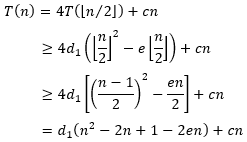现在要选取合适的 d 1 d_1 e e ，使得不等式 d 1 ( n 2 − 2 n + 1 − 2 e n ) + c n ≥ d 1 ( n 2 − e n ) d_1(n^2-2n+1-2en)+cn≥d_1(n^2-en) 成立。对该不等式做一下变换。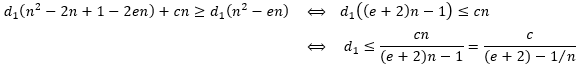当 n ≥ 2 n ≥ 2 时， c ( e + 2 ) − 1 / n \frac{c}{(e+2)-1/n} 是单调递减的，并且在 n = ∞ n = ∞ 时取得最小值为 c e + 2 \frac{c}{e+2} 。因此，只要取 d 1 ≤ c e + 2 d_1 ≤ \frac{c}{e+2} ，就能使得不等式 d 1 ( n 2 − 2 n + 1 − 2 e n ) + c n ≥ d 1 ( n 2 − e n ) d_1(n^2-2n+1-2en)+cn≥d_1(n^2-en) 成立，此时 T ( n ) ≥ d 1 ( n 2 − e n ) T(n)≥d_1(n^2-en) 成立。
综合考虑初始情况 T ( 1 ) T(1) ，我们最终要取 e < 1 e < 1 并且 d 1 ≤ m i n { 1 1 − e , c e + 2 } d_1 ≤ min\{\frac{1}{1−e}, \frac{c}{e+2}\} 。于是 T ( n ) ≥ d 1 ( n 2 − e n ) T(n)≥d_1(n^2-en) 得证。
(2) 证明存在正常数 d 2 d_2 e e ，使得 T ( n ) ≤ d 2 ( n 2 − e n ) T(n)≤d_2(n^2-en) 对足够大的 n n 都成立
取初始情况 T ( 1 ) = 1 T(1) = 1 。只要取 d 2 ≥ 1 / ( 1 − e ) d_2 ≥ 1/(1−e) 并且 e < 1 e < 1 ，就能使得 T ( 1 ) ≤ d 2 ( 1 2 − e • 1 ) = d 2 ( 1 − e ) T(1) ≤ d_2(1^2 − e•1) = d_2(1 − e) 成立。
现在考虑 n ≥ 2 n ≥ 2 的情况。假设 T ( n ) ≤ d 2 ( n 2 − e n ) T(n)≤d_2(n^2-en) 1 , 2 , … , n − 1 1, 2, …, n−1 都成立，于是有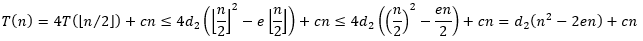现在要选取合适的 d 2 d_2 e e ，使得不等式 d 2 ( n 2 − 2 e n ) + c n ≤ d 2 ( n 2 − e n ) d_2(n^2-2en)+cn≤d_2(n^2-en) 成立。对该不等式做一下变换。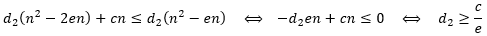因此，只要取 d 2 ≥ c / e d_2 ≥ c/e ，就能使能不等式 d 2 ( n 2 − 2 e n ) + c n ≤ d 2 ( n 2 − e n ) d_2(n^2-2en)+cn≤d_2(n^2-en) 成立，此时 T ( n ) ≤ d 2 ( n 2 − e n ) T(n)≤d_2(n^2-en) 成立。
综合考虑初始情况 T ( 1 ) T(1) ，我们最终要取 e < 1 e < 1 并且 d 2 ≤ m a x { 1 1 − e , c e } d_2 ≤ max\{\frac{1}{1−e}, \frac{c}{e}\} 。于是 T ( n ) ≤ d 2 ( n 2 − e n ) T(n)≤d_2(n^2-en) 得证。
综合(1)和(2)，只要取 e < 1 e < 1 d 1 ≤ m i n { 1 1 − e , c e + 2 } d_1 ≤ min\{\frac{1}{1−e}, \frac{c}{e+2}\} 并且 d 2 ≤ m a x { 1 1 − e , c e } d_2 ≤ max\{\frac{1}{1−e}, \frac{c}{e}\} ，就能使得 d 1 ( n 2 − e n ) ≤ T ( n ) ≤ d 2 ( n 2 − e n ) d_1(n^2-en)≤T(n)≤d_2(n^2-en) 成立。因此， T ( n ) = Θ ( n 2 ) T(n)=Θ(n^2) 得证。

4.4-8 对递归式 T ( n ) = T ( n − a ) + T ( a ) + c n T(n)=T(n-a)+T(a)+cn ，利用递归树给出一个渐近紧确解，其中 a ≥ 1 a ≥ 1 c > 0 c > 0 是常数。

创建递归树，如下图所示。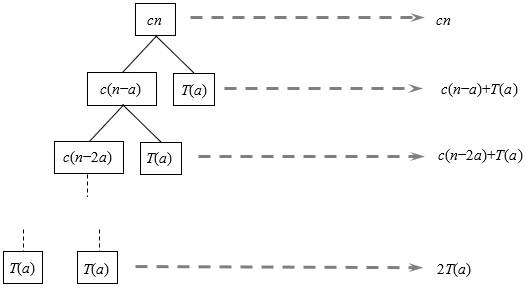在递归树中，每个结点均有 2 2 个孩子，其中右孩子都为 T ( a ) T(a) 。所以除根结点外，每一层都只有 2 2 个结点。深度为 i i 的结点其中一个对应规模为 n − a i n−ai 的子问题，另一个对应规模为 a a 的子问题。当 n − a i = a n − ai = a 时，即 i = ( n / a ) − 1 i = (n/a) − 1 时，子问题规模变为 a a ，这对应于最底层的叶结点 T ( a ) T(a) ，因此树的高度 h = ( n / a ) − 1 h = (n/a) − 1
除叶结点外，深度为 i i 的结点其中一个对应规模为 n − a i n−ai 的子问题，它的代价为 c ( n − a i ) c(n−ai) ；另一个对应规模为 a a 的子问题，它的代价为 T ( a ) T(a) 。因此，除叶结点外，深度为 i i 的所有结点的代价为 c ( n − a i ) + T ( a ) c(n−ai) + T(a) 。叶结点一共有 2 2 个，所以叶结点那一层的代价为 2 T ( a ) 2T(a)
现在将每一层的代价加起来，得到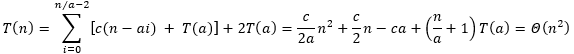4.4-9 对递归式 T ( n ) = T ( α n ) + T ( ( 1 − α ) n ) + c n T(n)=T(αn)+T((1-α)n)+cn ，利用递归树给出一个渐近紧确解，其中 0 < α < 1 0 < α < 1 c > 0 c > 0 是常数。

创建递归树，如下图所示。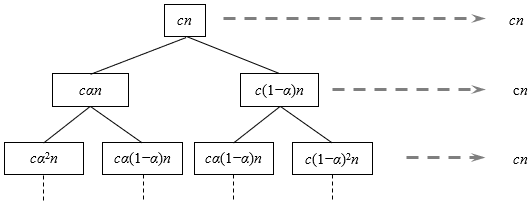为方便起见，假设 0 < α ≤ 1 / 2 0 < α ≤ 1/2 1 / 2 < α < 1 1/2 < α < 1 是对称的情况。从根结点到叶结点的最短简单路径是 n → α n → α 2 n → … → 1 n → αn → α2n → … → 1 。假设这一最短简单路径上叶结点深度为 k k ，那么有 α k • n = 1 α^k•n = 1 ，得到 k = l o g 1 / α n k={\rm log}_{1/α}n 。从根结点到叶结点的最短简单路径是 n → ( 1 − α ) n → ( 1 − α ) 2 n → … → 1 n → (1−α)n → (1−α)2n → … → 1 。假设这一最短简单路径上叶结点深度为 l l ，那么有 ( 1 − α ) l • n = 1 (1−α)^l•n = 1 ，得到 l = l o g 1 / ( 1 − α ) n l={\rm log}_{1/(1-α)} n
上图画出了递归树的顶部几层，顶部几层的代价都等于 c n cn ，严格来说，从根结点到第 l o g 1 / α n {\rm log}_{1/α}n 层，每一层的代价都为 c n cn 。只统计根结点到第 l o g 1 / α n {\rm log}_{1/α}n 层的代价，就可以得到 T ( n ) T(n) 的下界，于是有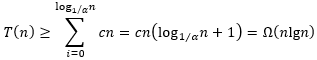随着递归树层级往下降，缺失的内部结点越来越多，因此底部几层的代价都不超过 c n cn 。于是有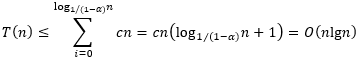综合以上分析，得到 T ( n ) = Θ ( n l g n ) T(n) = Θ(n{\rm lg}n)

展开全文算法导论
• 递归树和时间复杂度 递归算法的思想是将大问题分解为小问题，小问题再分解为小小问题，一直分解到足够小，不能分解为止。我们把这一层一层的分解过程画成一棵树，这个树就叫递归树。 以递归排序为例，分析一下递归...

递归树和时间复杂度
递归算法的思想是将大问题分解为小问题，小问题再分解为小小问题，一直分解到足够小，不能分解为止。我们把这一层一层的分解过程画成一棵树，这个树就叫递归树。

以递归排序为例，分析一下递归排序的时间复杂度。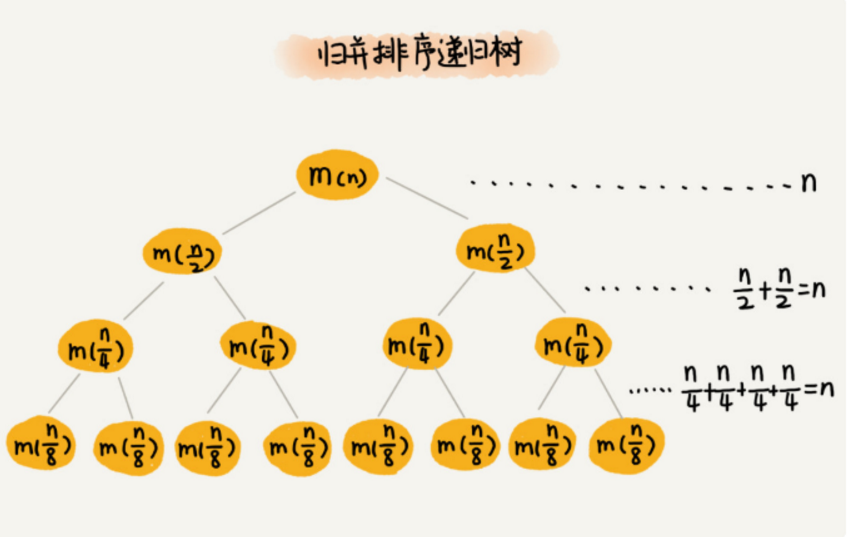从归并排序递归树我们发现，每一层的归并操作消耗的时间总和是一样的，都是n，那么只需要知道树的高度h，就可以算法时间复杂度O(n*h)。
这个递归树是一个满二叉树，而满二叉树的高度是logn，所以归并排序的时间复杂度是O(nlogn)。

快速排序的时间复杂度
快读排序最好情况下，每次分区都一分为二，根据上面介绍的，时间复杂度是O(nlogn)。但是当不是一分为二时时间复杂度是多少呢？例如，每次分区的比例为1:9。此时利用递归树计算时间复杂度。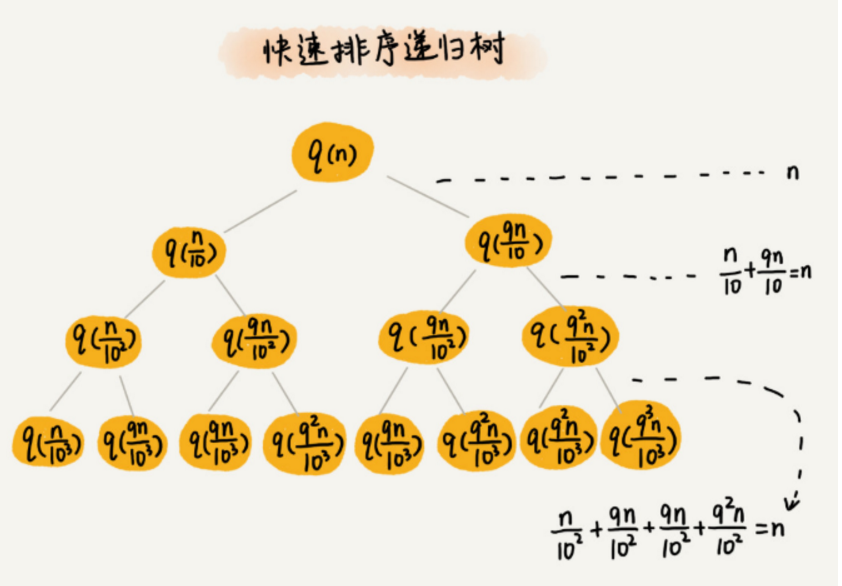同样每层遍历的数据之和都是n，只需要计算出树的高度h，就可以算的时间复杂度O(n*h)。
从递归树我们发现，最长路径是每次都乘9/10，最短路径是每次都乘1/10。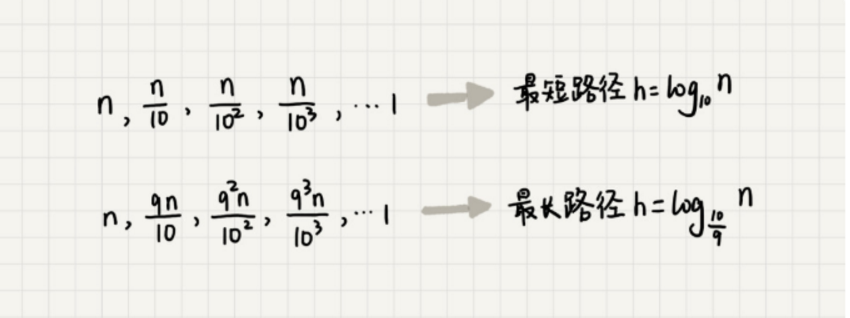不管是最长路径或者最短路径，时间复杂度都是O(nlogn)。

婓波那契数列的时间复杂度
斐波那切数列我们都知道，是这样的一个数列：

1、1、2、3、5、8、13、21、34、……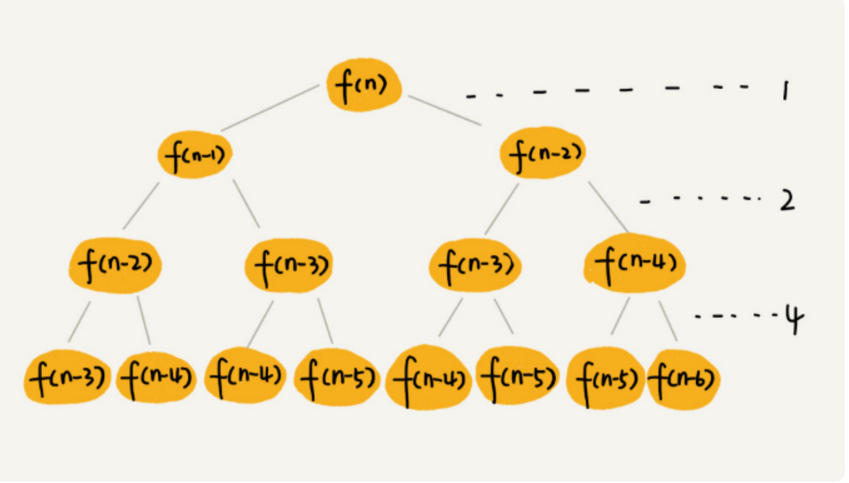f(n)=f(n-1)+f(n-2)，每次都是-1或者-2，所以最长路径是每次-1，长度是n；最短路径是每次-2，长度是n/2。
第一层需要消耗1一次运算，第二层消耗2次运算，第三层消耗4次运算，以此类推，第n层消耗2^(n-1)次运算。
当路径长度为n，则总和为当路径长度为n/2，则总和为所以时间复杂度为O(2n)和O(2n/2)之间。

原文：https://gper.club/articles/7e7e7f7ff7g54gcbg61

展开全文• 前言 我们都知道，递归代码的时间复杂度分析起来很麻烦。在排序那一节讲过，如何利用递推公式，求解归并排序和快速排序的时间复杂度，但是，有些情况下，比如...某些情况下，借助递归树可以快速分析递归算法的时间复...数据结构 算法
• 主要介绍了Java递归遍历形结构的相关资料,需要的朋友可以参考下
• ## 递归树求解递归方程

千次阅读 多人点赞 2021-07-31 09:56:08
首先了解一下这个递归式 T(n)=4T(n/2)+n 是什么意思： 4表示我们将一个问题分解为 4 个子问题 n/2表示每个子问题的规模是原问题的 1/2 n表示合并需要的额外计算时间 方法一可用主定理【Master定理】 主定理...
• ## 用递归树求解递归算法时间复杂度

万次阅读 多人点赞 2019-07-01 23:27:38
一般来说有两种分析方法：递推公式和递归树。 1 递推公式法 归并排序的递推公式是： merge_sort(p…r) = merge(merge_sort(p…q), merge_sort(q+1…r)) 终止条件： p >= r 不用再继续分解 我们假设对n个...时间复杂度
• 4.递归树 一、什么是递归树 如果我们把这个一层一层的分解过程画成图，它其实就是一棵树。我们给这棵树起一个名字，叫作递归树。 时间复杂度分析的递归树法 分析每一步核心操作的时间复杂度 分析树高：最大树高和...时间复杂度
• 递归树 使用p5js在JavaScript中以递归方式绘制的随机树。 在以下位置查看： : 例：
• 主要介绍了JavaScript实现无限级递归树的示例代码，文中通过示例代码介绍的非常详细，对大家的学习或者工作具有一定的参考学习价值，需要的朋友们下面随着小编来一起学习学习吧
• 前言 递归代码的时间复杂度分析起来很麻烦。《[数据结构与算法] 排序(三) 平均时间复杂度O(nlogn)》那里讲过，如何利用递推公式，求解归并排序、快速排序的时间复杂度，但是，有些情况，比如快...递归树与时间复杂...
• 那么什么是递归树？简单来说，这颗树是由递归的子问题组成树，树的节点就是递归的子问题。 比如斐波那契数列的递归树就是这个样子： 一个节点的求解可以分解为两个左右子节点的求解过程，就这样递归下去形成了...时间复杂度
• 27|递归树如何借助树来求解递归算法的时间复杂度 27|递归树如何借助树来求解递归算法的时间复杂度 今天我们来讲树这种数据结构的一种特殊应用递归树 我们都知道递归代码的时间复杂度分析起来很麻烦我们在第12节排序...
• ## 【算法设计与分析】11 递归树

千次阅读 多人点赞 2019-09-15 21:18:57
当前面所学习的迭代法、差消法等不太好解决的问题，可以使用递归树，来很方便的解决。 文章目录1. 递归树的概念1.1 迭代在递归树中的表示2. 递归树的生成规则2.1 递归树生成实例2.2 递归树应用实例3. 总结 1. 递归...
• 递归的思想就是，将大问题分解为...我们给这棵树起一个名字，叫作递归树。 如何用递归树求解时间复杂度 递归树分析归并排序时间复杂度 归并排序每次会将数据一分为二，因为每次分解都是一分为二，所以代价...
• mysql之递归树查询   写在前面   最近一段时间做的产品使用到了mysql数据库，虽然之前也使用过，但都是在业余时间使用，真正在项目中使用还是发现mysql的一些不同之处，比如函数、存储过程等，写起来还是比较...
• ## 用递归树方法求解递归式

千次阅读 多人点赞 2019-11-26 21:50:38
递归树方法求解递归式 递归式：T(n)=3T(n/4)+Θ(n2)T\left(n\right)=3T\left(n/4\right)+Θ\left(n^2\right)T(n)=3T(n/4)+Θ(n2) 我们先来了解一下这个递归式什么意思： 333 表示我们将一个问题分解为333个子...
• Java递归将List转为形结构 博客地址：https://blog.csdn.net/weixin_38500202/article/details/110456363
• 　在引入递归树之前可以考虑一个例子： 　T(n) = 2T(n/2) + n2 　迭代2次可以得： 　T(n) = n2+ 2(2T(n/4) + (n/2)2) 　还可以继续迭代，将其完全展开可得： 　T(n) = n2+ 2((n/2)2+ 2((n/22)2+ 2((n/23)2+ 2(...
• XDOJ 输出快速排序递归算法隐含递归树的后序遍历序列 点进来吧，不骗你算法 排序算法 c++
• ## js无限分类递归树

千次阅读 2021-11-16 17:57:45
首先了解一下什么是无限分类递归树（树形分类），如下示例： const arr = [ { id: 1, pid: 0, name: '1' }, { id: 2, pid: 0, name: '2' }, { id: 3, pid: 1, name: '3' }, { id: 4, pid: 3, name: '4' }, ...javascript
• 数据结构递归树.ppt该文档详细且完整，值得借鉴下载使用，欢迎下载使用，有问题可以第一时间联系作者~
• php利用递归实现分类显示（使用递归实现分类显示，要写好多字哟能不能少写点,复制几行凑数，使用递归实现分类显示使用递归实现分类显示使用递归实现分类显示）
• 今天，来讲树这种数据结构的一种特殊的应用，递归树。 我们都知道，递归代码的时间复杂度分析起来很麻烦，我们在排序（下）那里讲过，如何利用递推公式，求解归并排序的时间复杂度，但是，有此情况，比如快排的平均...
• 但往往前端所要的数据是直接返回，以便递归展示，即将每个节点下挂上孩子节点。 既然如此，各个语言均是互通的，博主仅提供python递归写法，请客官细品： class BTree(object): def __init__(self,tree,primary_...python...